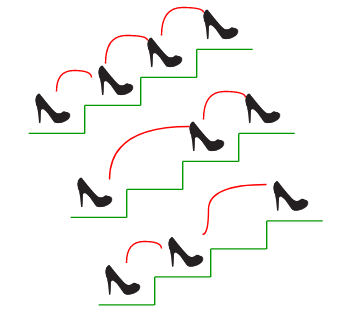Related Articles

# C# Program for Count ways to reach the n\’th stair

• Last Updated : 02 Jan, 2019

There are n stairs, a person standing at the bottom wants to reach the top. The person can climb either 1 stair or 2 stairs at a time. Count the number of ways, the person can reach the top.Consider the example shown in diagram. The value of n is 3. There are 3 ways to reach the top. The diagram is taken from Easier Fibonacci puzzles

## C#

 `// C# program to count the``// number of ways to reach``// n'th stair``using` `System;`` ` `class` `GFG {``    ``// A simple recursive``    ``// program to find n'th``    ``// fibonacci number``    ``static` `int` `fib(``int` `n)``    ``{``        ``if` `(n <= 1)``            ``return` `n;``        ``return` `fib(n - 1) + fib(n - 2);``    ``}`` ` `    ``// Returns number of ways``    ``// to reach s'th stair``    ``static` `int` `countWays(``int` `s)``    ``{``        ``return` `fib(s + 1);``    ``}`` ` `    ``// Driver Code``    ``static` `public` `void` `Main()``    ``{``        ``int` `s = 4;``        ``Console.WriteLine(``"Number of ways = "` `+ countWays(s));``    ``}``}`` ` `// This code is contributed``// by akt_mit`
Output:
```Number of ways = 5
```

The time complexity of the above implementation is exponential (golden ratio raised to power n). It can be optimized to work in O(Logn) time using the previously discussed Fibonacci function optimizations.

## C#

 `// C# program to Count ways to reach``// the n’th stair``using` `System;`` ` `class` `GFG {`` ` `    ``// A recursive function used by``    ``// countWays``    ``static` `int` `countWaysUtil(``int` `n, ``int` `m)``    ``{``        ``if` `(n <= 1)``            ``return` `n;``        ``int` `res = 0;`` ` `        ``for` `(``int` `i = 1; i <= m && i <= n; i++)``            ``res += countWaysUtil(n - i, m);``        ``return` `res;``    ``}`` ` `    ``// Returns number of ways to reach``    ``// s'th stair``    ``static` `int` `countWays(``int` `s, ``int` `m)``    ``{``        ``return` `countWaysUtil(s + 1, m);``    ``}`` ` `    ``/* Driver program to test above function */``    ``public` `static` `void` `Main()``    ``{``        ``int` `s = 4, m = 2;``        ``Console.Write(``"Number of ways = "``                      ``+ countWays(s, m));``    ``}``}`` ` `// This code is contributed by nitin mittal.`
Output:
```Number of ways = 5
```

## C#

 `// C# program to count number``// of ways to reach n't stair when``// a person can climb 1, 2, ..m``// stairs at a time``using` `System;``class` `GFG {`` ` `    ``// A recursive function``    ``// used by countWays``    ``static` `int` `countWaysUtil(``int` `n, ``int` `m)``    ``{``        ``int``[] res = ``new` `int``[n];``        ``res = 1;``        ``res = 1;``        ``for` `(``int` `i = 2; i < n; i++) {``            ``res[i] = 0;``            ``for` `(``int` `j = 1; j <= m && j <= i; j++)``                ``res[i] += res[i - j];``        ``}``        ``return` `res[n - 1];``    ``}`` ` `    ``// Returns number of ways``    ``// to reach s'th stair``    ``static` `int` `countWays(``int` `s, ``int` `m)``    ``{``        ``return` `countWaysUtil(s + 1, m);``    ``}`` ` `    ``// Driver Code``    ``public` `static` `void` `Main()``    ``{``        ``int` `s = 4, m = 2;``        ``Console.WriteLine(``"Number of ways = "` `+ countWays(s, m));``    ``}``}`` ` `// This code is contributed by anuj_67.`
Output:
```Number of ways = 5
```

Please refer complete article on Count ways to reach the n’th stair for more details!

My Personal Notes arrow_drop_up